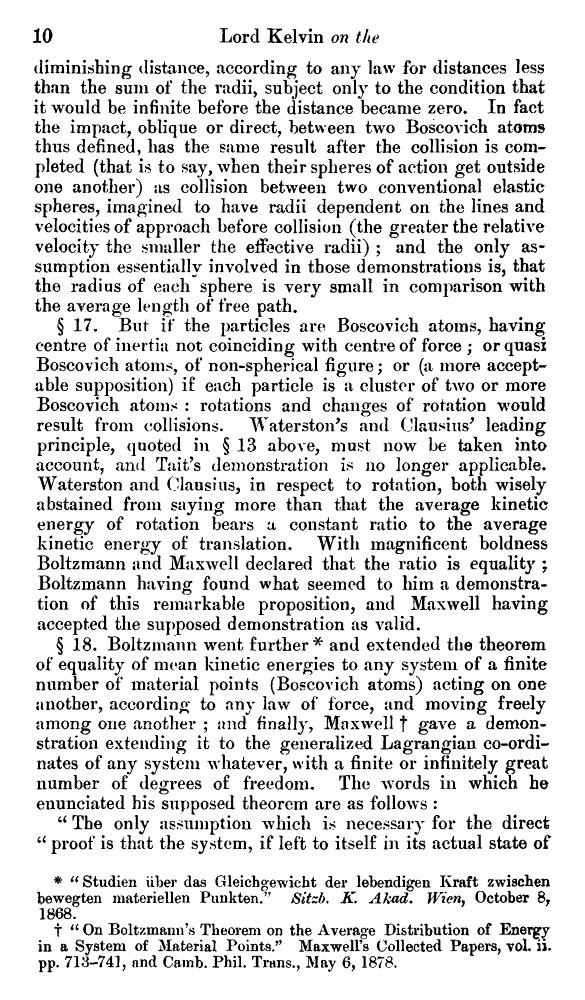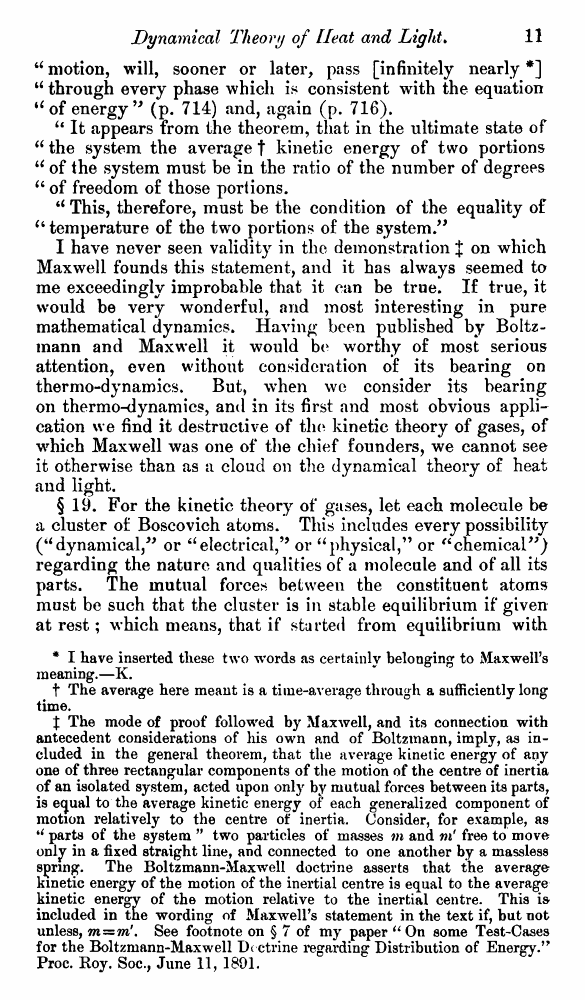﻿ ﻿ Lord Kelvin. Nineteenth-Century Clouds over the Dynamical Theory of Heat and Light. // Phil. Mag. S. 6. Vol. 2. No. 7. July 1901.

# Lord Kelvin. Nineteenth-Century Clouds over the Dynamical Theory of Heat and Light. // Phil. Mag. S. 6. Vol. 2. No. 7. July 1901.

В начало   Другие форматы   <<<     Страница 10   >>>1  2  3  4  5  6  7  8  9  10 11  12  13  14  15  16  17  18  19  20  21  22  23  24  25  26  27  28  29  30  31  32  33  34  35  36  37  38  39  40 diminishing distance, according to any law for distances less than the sum of the radii, subject only to the condition that it would be infinite before the distance became zero. In fact the impact, oblique or direct, between two Boscovich atoms thus defined, has the same result after the collision is completed (that is to say, when their spheres of action get outside one another) as collision between two conventional elastic spheres, imagined to have radii dependent on the lines and velocities of approach before collision (the greater the relative velocity the smaller the effective radii) ; and the only assumption essentially involved in those demonstrations is, that the radius of each sphere is very small in comparison with the average length of free path. § 17. But if the particles are Boscovich atoms, having centre of inertia not coinciding with centre of force ; or quasi Boscovich atoms, of non-spherical figure; or (a more acceptable supposition) if each particle is a cluster of two or more Boscovich atoms : rotations and changes of rotation would result from collisions. Waterston's and Clausius* leading principle, quoted in § 13 above, must now be taken into account, and Tait’s demonstration is no longer applicable. Waterston and Clausius, in respect to rotation, both wisely abstained from saying more than that the average kinetic energy of rotation bears a constant ratio to the average kinetic energy of translation. With magnificent boldness Boltzmann and Maxwell declared that the ratio is equality ; Boltzmann having found what seemed to him a demonstration of this remarkable proposition, and Maxwell having accepted the supposed demonstration as valid. § 18. Boltzmann went further* and extended the theorem of equality of mean kinetic energies to any system of a finite number of* material points (Boscovich atoms) acting on one another, according to any law of force, and moving freely among one another ; and finally', Maxwell t gave a demonstration extending it to the generalized Lagrangian co-ordinates of any system whatever, with a finite or infinitely great number of degrees of freedom. The words in which he enunciated his supposed theorem are as follows : u The only assumption which is necessary for the direct u proof is that the system, if left to itself in its actual state of * “Studien liber das Gleichgewicht der lebendigen Kraft zwischen bewegten materiellen Punkten.” Sitzb. K. Akad. Wieny October 8, 1868. t u On Boltzmann’s Theorem on the Average Distribution of Energry in a System of Material Points/* Maxwell’s Collected Papers, vol. ii. pp. 713-741, and Camb. Phil. Trans., May 6, 1878. “ motion, will, sooner or later, pass [infinitely nearly *] “ through every phase which is consistent with the equation “of energy ” (p. 714) and, again (p. 716). u It appears from the theorem, that in the ultimate state of 66 the system the average t kinetic energy of two portions u of the system must be in the ratio of the number of degrees “ of freedom of those portions. u This, therefore, must be the condition of the equality of “ temperature of the two portions of the system.” I have never seen validity in the demonstration J on which Maxwell founds this statement, and it has always seemed to me exceedingly improbable that it can be true. If true, it would be very wonderful, and most interesting in pure mathematical dynamics. Having been published by Boltzmann and Maxwell it would be worthy of most serious attention, even without consideration of its bearing on thermo-dynamics. But, when we consider its bearing on thermo-dynamics, and in its first and most obvious application we find it destructive of the kinetic theory of gases, of wrhich Maxwell was one of the chief founders, we cannot see it otherwise than as a cloud on the dynamical theory of heat and light. § 19. For the kinetic theory of gases, let each molecule be a cluster of Boscovich atoms. This includes every possibility (“dynamical,” or “electrical,199 or “physical,” or “chemical”) regarding the nature and qualities of a molecule and of all its parts. The mutual forces between the constituent atoms must be such that the cluster is in stable equilibrium if given at rest; which means, that if started from equilibrium with * I have inserted these two words as certainly belonging to Maxwell’s meaning.—K. t The average here meant is a time-average through a sufficiently long time. % The mode of proof followed by Maxwell, and its connection with antecedent considerations of his own and of Boltzmann, imply, as included in the general theorem, that the average kinetic energy of any one of three rectangular components of the motion of the centre of inertia of an isolated system, acted upon only by mutual forces between its parts, is equal to the average kinetic energy of each generalized component of motion relatively to the centre of inertia. Consider, for example, as ** parts of the system ” two particles of masses m and m' free to move only in a fixed straight line, and connected to one another by a massless spring. The Boltzmann-Maxwell doctrine asserts that the average kinetic energy of the motion of the inertial centre is equal to the average kinetic energy of the motion relative to the inertial centre. This is-included in the wording of Maxwell’s statement in the text if, but not unless, mz=m'. See footnote on § 7 of my paper “ On some Test-Cases for the Boltzmann-Maxwell Dcctrine regarding Distribution of Energy.” Proc. Roy. Soc., June 11, 1801.